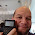## Monday, November 21, 2011

### HP 15C Programming Tutorial - Part 8: The Summation Program

Sum

This program computes the sum:

R0 = ∑( X = R1, R2, f(R1))

where:
R0 = the sum
R1 = starting value, which gets updated at each step. R1 is also used as the variable for f(X)
R2 = finishing value

The program uses three labels:

Label A: The main routine. Press [ f ] [ √ ] (A) to run the routine.
Label 1: A subroutine of Label A. This is the loop.
Label 0: Where the function f(X) is stored. Assume that R1 is loaded on the X register when programming the function.

The program presented here will allow the user to enter starting and ending values beyond 999. You can shorten the program by the use of ISG, however, the ending value would be restricted to 999.

Program Listing

`Key Code			Keys001	42	21	11	LBL A002			0	0003		44	0	STO 0	* Initializes R0004			33	R ↓ 005		44	2	STO 2	* ending value006			33	R ↓007		44	1	STO 1	* starting value008	42	21	1	LBL 1	* loop starts here009		45	1	RCL 1010		32	0	GSB 0011	44	40	0	STO+ 0	* update total012			1	1013	44	40	1	STO+ 1	* update starting value014		45	2	RCL 2015		45	1	RCL 1016		43	10	x≤y	* is R1 ≤ R2?017		22	1	GTO 1	018		45	0	RCL 0019		43	32	RTN`

Instructions:
1. Enter the main program (Labels A and 1).
2. Enter the function f(R1) (Label 0).
3. In run mode, enter the starting value and press [ENTER]
4. Enter the ending value and press [ f ] [ √ ] (A)
5. The sum is calculated and displayed.

Remember: Always finish the function with the RTN command ([ g ] [GSB] (RTN)).

Example 1

Find the sum ∑(X = 1, 50, X). In other words, what is the sum of the integers from 1 to 50?

Program listing for f(R1):

`Key Code		Key001	42	21	0	LBL 0002		43	32	RTN`

Surprised? Remember we already have a copy of R1 in the main program when the instruction GSB 0 is encountered.

1 [ENTER] 50 [ f ] [ √ ] (A)

Result: 1275

Example 2

Find the sum ∑(X = 1, 150, 1/(X^2 + X)).

1/(X^2 + X) can be rewritten as:

(X^2 + X)^-1

((X + 1) X)^-1

We will use the last form for our function.

A Way to Clear Label 0

Clear Label 0 is necessary. In Run mode, press [GTO] [ 0 ]. Then press [ g ] [R/S] (P/R) to enter program mode. Press [SST] until the key codes "43 32" is encountered. Press the backspace button the number of times you pressed [SST]. You should see the key code "42, 21, 0". You are ready to enter the new function.

Program Listing for f(R1):
`Key Code		Key001	42	21	0	LBL 0002			36	ENTER003			1	1004			40	+005			20	×006			15	1/x007		43	32	RTN`

To finish:

1 [ENTER] 150 [ f ] [ √ ] (A)

Result: ≈ 0.9934

That concludes Part 8 of our tutorial. Until next time,

Eddie

This tutorial is property of Edward Shore. © 2011

1.Hello Eddie, is there any way to enter "infinite" as the value of R2 in your program?

For example, if I want to evaluate ∑(X = 0, infinite, (1/4)^x).

Label 0 would be:
001 Label 0
002 Enter
003 1
004 Enter
005 4
006 /
007 x<>Y
008 Y^x
009 RTN

2.P.C.F.:

No, there is no infinity key on the HP-15C. We can modify the program a bit (as long as f(x) approaches 0 as x approaches infinity), to a specific tolerance. In this example, the tolerance is 10^-12. The program stops the first time f(x_n) < 10^-12:

LBL A
0
STO 0
STO 1
LBL 1
RCL 1
GSB 0
STO+ 0
EEX
1
2
CHS
x>y? (TEST 7)
GTO 2
1
STO+ 1
GTO 1
LBL 2
RCL 0
RTN

LBL 0
1
ENTER
4
ENTER
/
x<>y
y^x
RTN

Another way to do add to infinity to set an artificially high number for R2 (such as 500 or 1000).

Hope this helps,

Eddie

3.Hi Eddie, thank you so much for your quick reply I really appreciate it. The modified program works fine and so does the second way you provided. Really helps me out.

### Σ(1 / (a^n)) from n=1 to m

Σ(1 / (a^n)) from n=1 to m This blog entry covers the sum of the series: Σ[1 / (a^n), n=1 to m] with n and m positive integers Specific Cas...